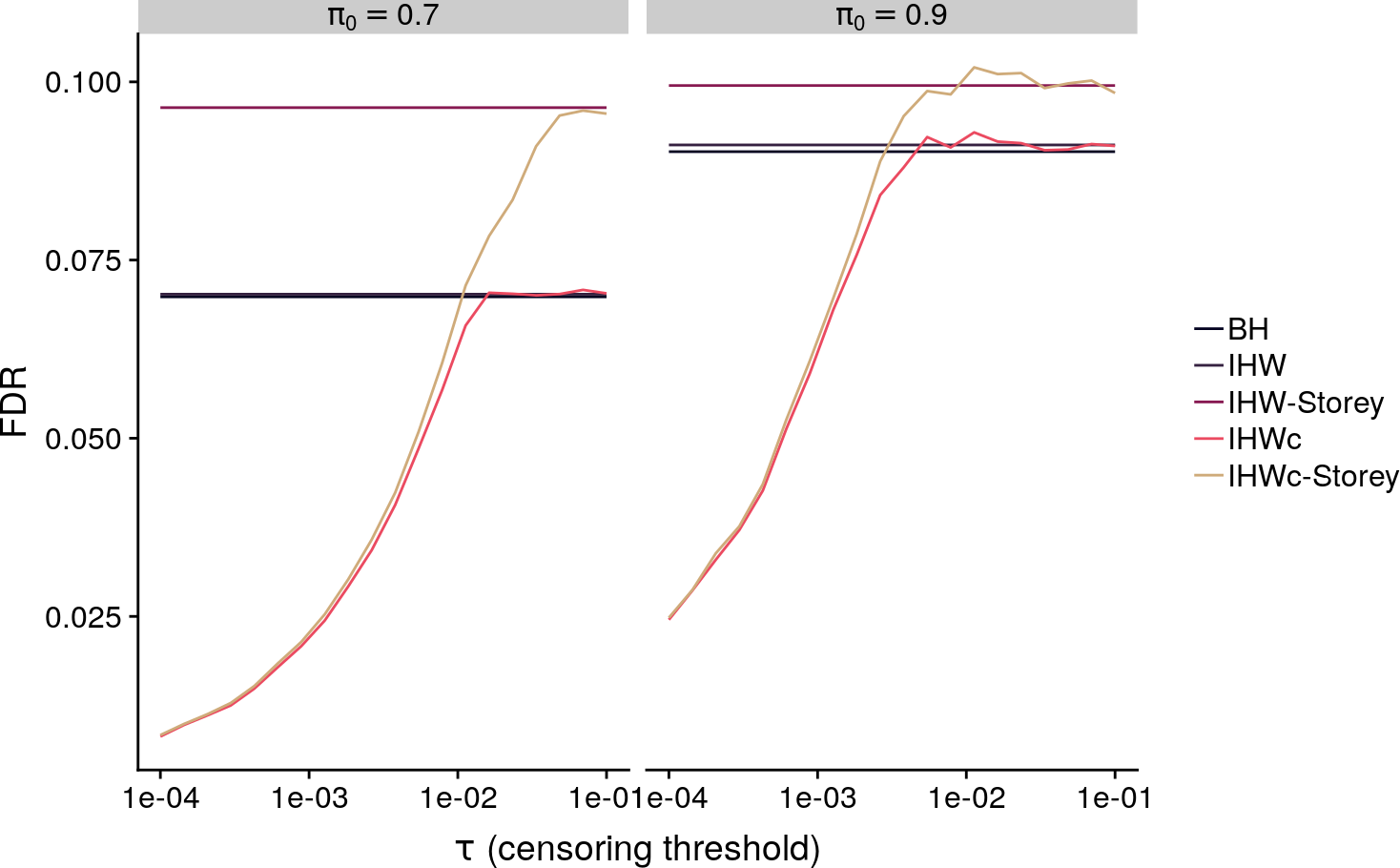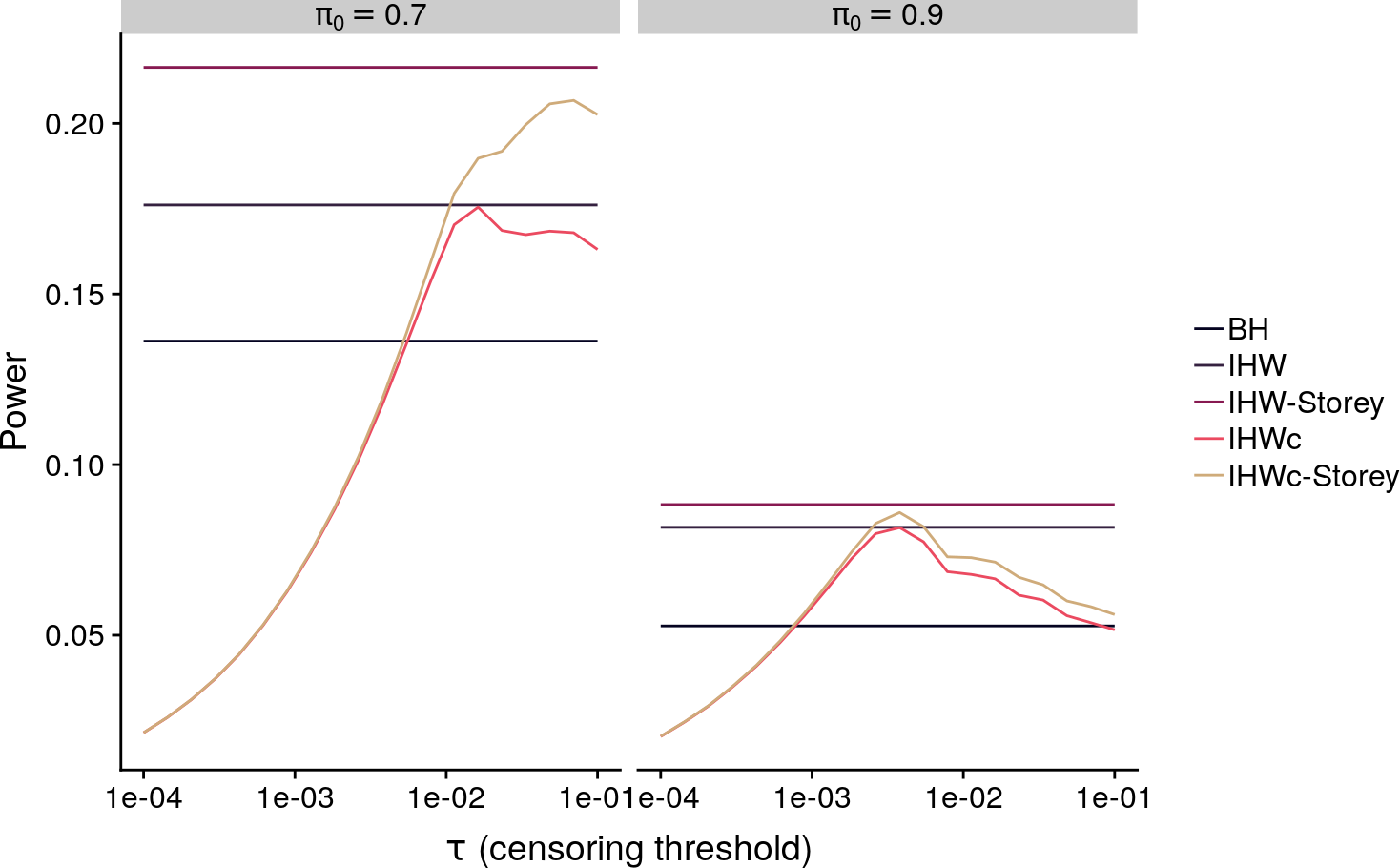1 Simulation setting and methods compared

As IHW has been benchmarked thoroughly elsewhere (for example, the rest of this package contains many such simulations), here we restrict ourselves to a small study to illustrate the effects of censoring (as the censoring threshold $$\tau$$ varies) and null-proportion adaptivity.

In particular, we consider the following example of a simple conditional two-groups model (for two different values of $$\pi_0 \in \{0.7, 0.9\})$$:

\begin{aligned} &X_i \sim U[0,2.5]\\ &H_i \mid X_i \sim \text{Bernoulli}(\pi_0)\\ &Z_i \mid (H_i = 0, X_i) \sim \mathcal{N}(0,1)\\ &Z_i \mid (H_i = 1, X_i) \sim \mathcal{N}(X_i,1)\\ &P_i = 1 - \Phi(X_i) \end{aligned} We compare the following approaches, using the nominal level $$\alpha=0.1$$, $$P_i$$ as the p-values and $$X_i$$ as the covariates (except for BH which does not account for covariates):

1. BH (Benjamini and Hochberg 1995)
2. IHW
3. IHW with Storey $$\pi_0$$ correction ($$\tau'=0.5$$)
4. IHWc with different censoring thresholds $$\tau$$
5. IHWc with Storey $$\pi_0$$ correction ($$\tau'=0.5$$) and different censoring thresholds $$\tau$$.

The censored IHW (IHWc) variant was implemented as follows:

1. We censor the p-values, i.e. we set all p-values below $$\tau$$ to 0.
2. As in classical IHW, we discretize the covariate into a finite number of strata (20) and also randomly split the hypotheses into 5 folds.
3. Within each combination of stratum and fold, we fit the Beta-Uniform model with the EM-method of (Markitsis and Lai 2010), which can take censoring into account.
4. For each censored p-value, we draw a new p-value from its fitted Beta-Uniform model conditionally on it being $$\leq \tau$$, i.e. we impute all censored p-values.
5. Then we use regular IHW to learn the weight functions.
6. We use weighted BH after cross-weighting the original (i.e. non-censored/non-imputed) p-values, making sure we do not reject any p-value $$\leq \tau$$.

For the exact details of the simulation and also to reproduce it, see the folder “inst/theory_paper/ihwc_wasserman_normal_simulation.R” of this package.

2 Simulation results

library("ggplot2")
library("grid")
library("dplyr")
library("tidyr")
library("cowplot")
library("IHWpaper")
# color from https://github.com/dill/beyonce
# beyonce::beyonce_palette(30,5)
colors <- c("#040320", "#352140", "#871951", "#EB4A60", "#CFAB7A")
sim_folder <- system.file("simulation_benchmarks/theory_paper",
package = "IHWpaper")
sim_df <- readRDS(file.path(sim_folder, "ihwc_wasserman_normal_sim.Rds"))
taus_df <- expand.grid(fdr_pars = unique(sim_df$fdr_pars), fdr_method = c("BH","IHW","IHW-Storey"), sim_pars = unique(sim_df$sim_pars)) %>%
na.exclude()

df1 <- left_join(taus_df, select(sim_df, -fdr_pars), by=c("fdr_method","sim_pars"))  %>%
select(-fdr_pars.y) %>% rename(fdr_pars = fdr_pars.x)
## Warning: Detecting old grouped_df format, replacing vars attribute by
## groups
## Adding missing grouping variables: fdr_pars
## Warning: Column fdr_method joining factor and character vector, coercing
## into character vector
## Warning: Column sim_pars joining factor and character vector, coercing into
## character vector
df <- full_join(df1, filter(sim_df, fdr_method %in% c("IHWc_Storey","IHWc"))) %>%
mutate( fdr_method = ifelse(fdr_method=="IHWc_Storey","IHWc-Storey", fdr_method))
## Joining, by = c("fdr_pars", "fdr_method", "sim_pars", "alpha", "FDR", "power", "rj_ratio", "FPR", "FDR_sd", "FWER", "nsuccessful", "sim_method", "m")
pi0s <- sapply(strsplit(df$sim_pars, split="[[:punct:]]"), function(x) paste0(x[],".",x[])) df$pi0s <- pi0s
fdr_plot <- ggplot(df, aes(x=fdr_pars, y=FDR, col=fdr_method)) + geom_line() +
facet_grid(.~pi0s, labeller=label_bquote(cols=pi == .(pi0s))) +
scale_x_log10() +
scale_color_manual(values=colors) +
theme(legend.title=element_blank()) +
xlab(expression(tau~"(censoring threshold)")) +
ylab("FDR")
fdr_plotpower_plot <- ggplot(df, aes(x=fdr_pars, y=power, col=fdr_method)) + geom_line() +
facet_grid(.~pi0s, labeller=label_bquote(cols=pi == .(pi0s))) +
scale_x_log10() +
scale_color_manual(values=colors) +
theme(legend.title=element_blank()) +
xlab(expression(tau~"(censoring threshold)")) +
ylab("Power")
power_plot3 Discussion of simulation results

The first of the above figures shows the FDR of the procedures as $$\tau$$ varies (note that BH/IHW/IHW-Storey do not depend on $$\tau$$). Formally, only IHWc and IHWc-Storey have provable finite-sample control. However, we see that all methods are below the nominal $$\alpha=0.1$$. Furthermore, IHW-Storey is at essentially exactly $$0.1$$, i.e. by estimating $$\pi_0$$ it controls FDR at exactly the nominal level. This is also the case for IHWc-Storey when it is properly tuned. IHWc and IHWc can have FDR way below $$\alpha$$ for improper choice of the tuning parameter $$\tau$$.

The second plot shows the power of the procedures (defined as the expected proportion of true discoveries among all false null hypotheses). Of course for $$\pi_0 = 0.7$$ all methods have a lot more power than for $$\pi_0=0.9$$. We also observe the theoretically expected trade-off for the choice of censoring threshold $$\tau$$: If it is chosen way too large, then we cannot estimate the underlying conditional two-groups model well, thus the weights are suboptimal and we lose power. On the other hand, if $$\tau$$ is too small, then we lose a lot of power since we cannot reject the p-values below $$\tau$$. BH has a lot less power compared to IHW/IHW-Storey and also IHWc/IHWc-Storey for a wide range of censoring thresholds $$\tau$$.